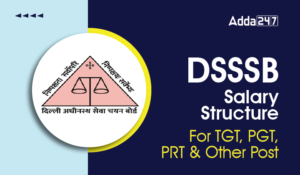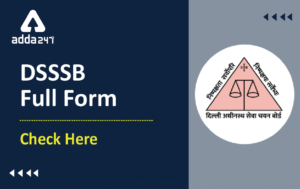Latest Teaching jobs   »   Reasoning Questions For DSSSB/HSSC Exam |...

# Reasoning Questions For DSSSB/HSSC Exam | 19th October 2019The reasoning is the most scoring section in any competitive exam. It also develops or nourishes our critical thinking skills as compared to other subjects. In DSSSBKVSAPS Exam as a student to score well in this part, you have to start your preparation now because there is negative marking as well. The more you have prepared, the better you score.

Q1. Odometer is to mileage as compass is to
(a) Speed
(b) Hiking
(c) Needle
(d) Direction

Q2. Yard is to inch as Quart is to
(a) Gallon
(b) Ounce
(c) Milk
(d) liquid

Q3. What will come in place of (?) question mark?
Candle   Lamp        Floodlight
Hut        Cottage         ?
(a) tent
(b) city
(c) dwelling
(d) house

Q4. If 25-2=3, 36-2=4, 49-2=5 then what is 64-2=?
(a) 4
(b) 5
(c) 6
(d) 7

Q5. Choose the odd one out
(a) A B B C
(b) P Q Q R
(c) H I I J
(d) W Y Y Z

Q6. If ORANGE = 60, GRAPES =?
(a) 61
(b) 65
(c) 64
(d) 66

Q7. If ROPE is written as TQRG, how is PURE written in this code?
(a) QVSF
(b) RWTG
(c) NSPC
(d) AQUA

Q8. CMM, EOO, GQQ, ________, KUU
(a) GRR
(b) GSS
(c) ISS
(d) ITT

Q9. How many degrees are there between two hands of a clock at 6:30 am?
(a) 0
(b) 15
(c) 10
(d) 30

Q10. One third of a number is four times eleven. What is half of the number?
(a) 22
(b) 36
(c) 66
(d) 44

Solutions

S1. Ans.(d)
Sol. Odometer is used to measure speed. Similarly, Compass is used to detect direction.

S2. Ans.(b)
Sol.  Yard and inches is unit for measuring length where Yard is more than inch. Similarly, quart and ounce is unit of measuring liquids where Quarts is more than ounce.

S3. Ans.(d)
Sol. Candle<Lamp<Flood light
Similarly, Hut<Cottage<House

S4. Ans.(c)
Sol.S5. Ans.(d)
Sol.S6. Ans.(d)
Sol.
O R A N G E

15+18+1+14+7+5 = 60
Similarly,

G R A P E S

7+18+1+16+5+19 = 66

S7. Ans.(b)
Sol.S8. Ans.(C)
Sol.S9. Ans.(b)
Sol.The total degrees in 12 hours = 360°
Degrees in 1 hour = (360°)/2=30°
Therefore degrees in 1/2  hour=30/2=15°

S10. Ans.(c)
Sol.  Suppose the number is x, then
x/3=4×11 ⟹x=132
So,x/2=132/2=66

You may also like to read :

Sharing is caring!

Thank You, Your details have been submitted we will get back to you.

### TOPICS:

•Delhi Guest Teacher Vacancy 2023 Out for...
•DSSSB Eligibility 2023 Check Post-Wise E...
•DSSSB Salary Structure 2023 in Hand Sala...
•DSSSB To Release 12,796 PRT, TGT Vacanci...
•DSSSB Full Form, Delhi Subordinate Servi...
•DSSSB Syllabus 2023 & Exam Pattern F...